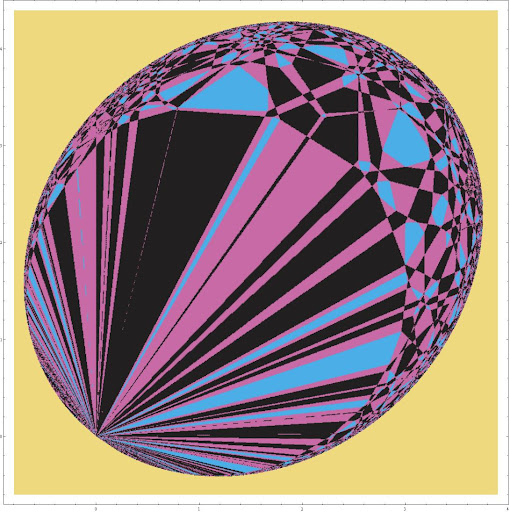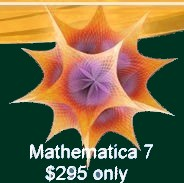## Monday, February 23, 2009

### Dualities vs singularities

A decade ago, when we wrote Dualities vs Singularities (PDF), I obviously had to be afraid of Mathematica. Otherwise I would have easily drawn diagrams similar to the following:Click to zoom in. It is kind of pretty. But what does it show? I must tell you a few words.

Take the 11-dimensional M-theory and compactify it on a rectangular k-torus with no C-field. The shape of the torus is described by "k" radii,
{exp(p1), exp(p2), ..., exp(pk)}
times the Planck length (or more precisely, the self-dual radius under a U-duality described later). It is useful to write the radii as exponentials because the logarithms, "p_i", behave linearly under the multiplication of the radii and their powers.

And when you perform U-dualities, there's a lot of multiplication of radii by powers of other (or the same) radii. The "p_i" coordinates naturally go from "-infinity" to "+infinity".The question was which values of the vectors "p" describe a physical situation whose rough behavior is well understood and where we have a natural perturbative expansion. For M-theory on tori, there are only three such descriptions:
• M-theory: on a large torus in Planck units (11D SUGRA etc.): BLUE
• Type IIA string theory: weakly coupled on a large torus in string units: PURPLE
• Type IIB string theory: weakly coupled on a large torus in string units: BLACK
Various inequalities for linear functions of "p_i" describe which conditions have to be satisfied for you to have each of these descriptions. Permutations of "p_i" don't matter. For example, if the smallest "p_i" is (still) positive, then you are in the blue M-theoretical region.

But the most important operation we can use are dualities. An elementary U-duality changes three radii by some linear transformation and the remaining ones by another transformation.

If you interpret one of the three special circles as the 11th coordinate added to a type IIA theory to obtain M-theory, this elementary U-duality may be interpreted as a double T-duality in type IIA string theory reflecting the other two circles, permuting them, and returning you to another version of type IIA string theory - that you subsequently reinterpret as a copy of M-theory again.
Download a Mathematica notebook drawing similar pictures
It can be seen that these permutations and U-dualities generate the Weyl group of "E_k". Yes, the exceptional groups are automatically incorporated in this simple linear algebra. The paper above also contains a simple proof why the M/IIA/IIB regions are non-overlapping and cover the whole parameter space of "p_i" when "k" is less than nine.

For "k=9", one half of the parameter space is understood - if the volume of the nine-torus is greater than the Planckian volume. For "k=9" or greater, there is no real physical moduli space because the scalar fields have huge, infrared fluctuations in the remaining 1+1 dimensions or less. But you can still calculate the action of U-dualities.

For "k=10", i.e. for the full cosmological compactification of all spatial dimensions, things become even more interesting because the ten-dimensional parameter space has a natural Lorentzian metric on it.

The picture above describes a rather random two-dimensional flat section through the ten-dimensional space. Because the ten-dimensional space contains a light-cone in it and the interior of the future light cone is exactly the big region that is understood, you see blue, purple, and black regions filling the interior of an ellipse. The yellow exterior is mysterious and corresponds to no known description.

You should notice that the (blue) M-theoretical regions are never adjacent to the (black) F-theoretical regions of type IIB string theory (by sharing a boundary): there are always (purple) type IIA regions in between. You should imagine the M-theoretical regions to be female, the F-theoretical regions are male, and the type IIA regions interpolate between them and they are gender-neutral.

Annoyingly enough, in English, "male" and "female" start with "M/F", while the corresponding parents, "father" and "mother", start with "F/M", so I decided not to use letters in the graph. :-) All three genders, female, male, and neutral, cover comparable portions of the moduli space.